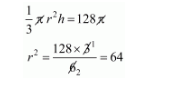# A cylinder with base radius of 8 cm and height of 2 cm`
Question:

A cylinder with base radius of 8 cm and height of 2 cm is melted to form a cone of height 6 cm. The radius of the cone is

(a) 4 cm

(b) 5 cm

(c) 6 cm

(d) 8 cm

Solution:

Volume of cylinder $=\pi r^{2} h=\pi \times(8)^{2} \times 2=128 \pi \mathrm{cm}^{2}$

Let $r$ be the radius of cone

But,

The volume of cone $=$ volume of cylinder$r=8 \mathrm{~cm}$

Hence, Radius of cone = 8 cm.

Hence, the correct answer is choice (d).